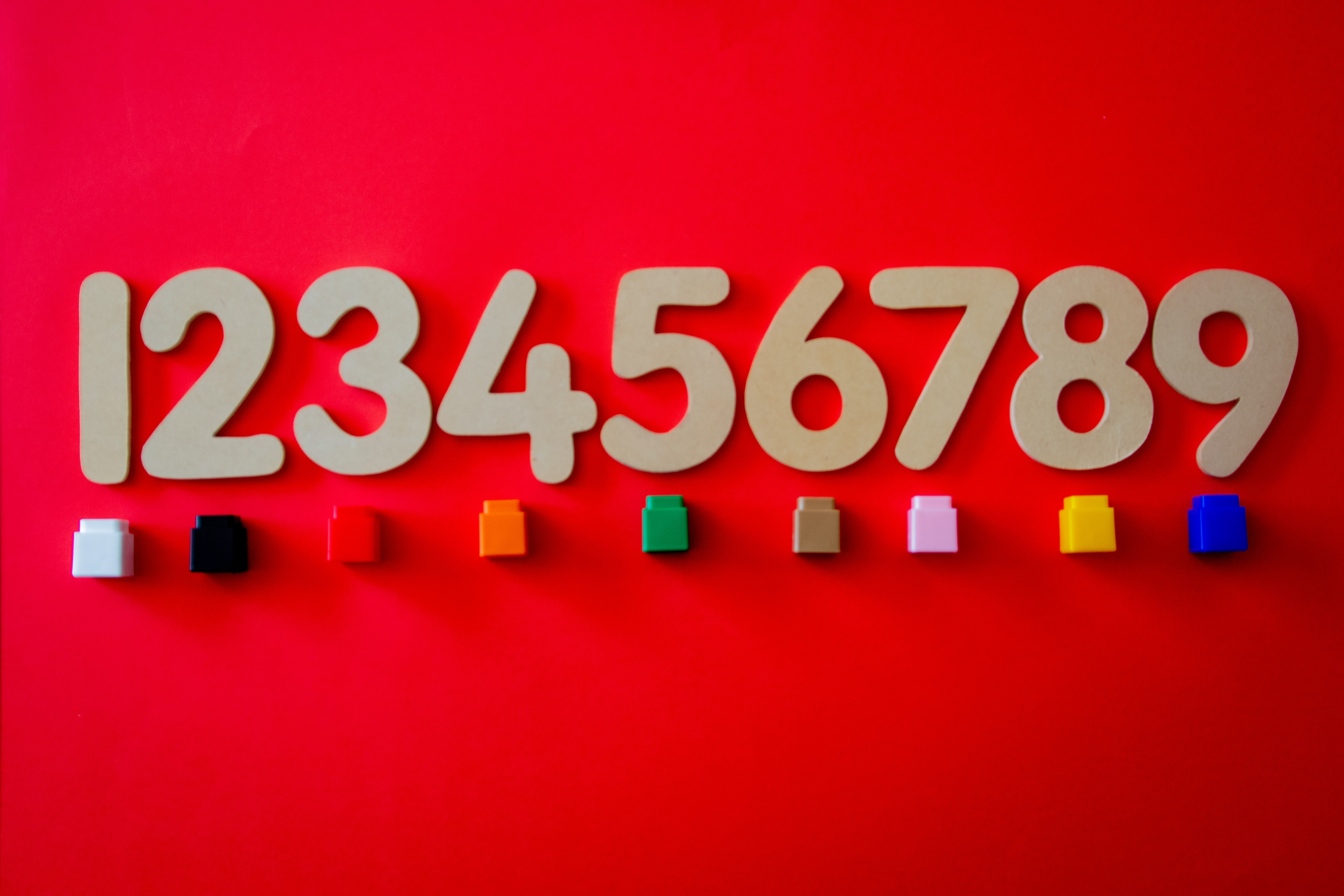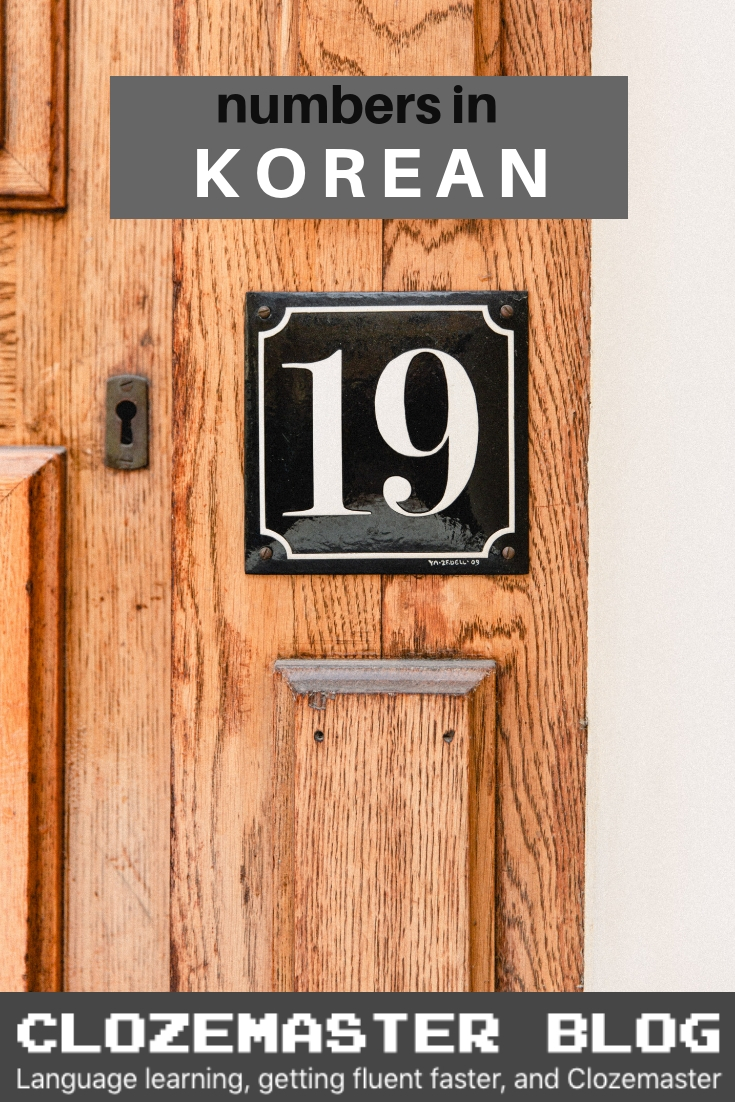Blog » Language Learning » Learn Korean » A Crash Course in Korean Numbers

#Learning Korean numbers requires a little more attention than learning numbers in most other languages. This is because unlike most other languages, the Korean language has two number systems. One number system is called Sino-Korean numbers, and this is a number system based on Chinese characters, and the other is called native Korean numbers, and as the name of the number system would suggest, this is based on Korean language.

When learning Korean numbers, you do have to learn both number systems because each has particular uses in Korean, so you can’t just learn on and use that for everything – that would sound very unnatural.

Fortunately, although there are two different systems, they’re both quite easy to master, so in this article, we’ll learn how these number systems work, and we’ll also take a look at how they’re used in Korean.

## Sino-Korean numbers

Because Koreans used Chinese characters as a writing system before the creation of Hangul, much of Korean language is heavily influenced by Chinese language, and Korean numbers is one of the most influenced aspects.

Fortunately, although the numbers are based on Chinese characters, you won’t have to learn any Chinese characters – you can just learn how they’re written in Hangul.

### 0 to 10

Now, the key to learning Sino-Korean numbers is all about mastering the numbers 0 to 10, and you’ll soon find out why.

• 0 – (young)
• 1 – (il)
• 2 – (ee)
• 3 – (sam)
• 4 – (sa)
• 5 – (oh)
• 6 – (yook)
• 7 – (chil)
• 8 – (pal)
• 9 – (gu)
• 10 – (ship)

### 11 to 99

To form double-digit numbers using Sino-Korean numbers, you just have to combine the number words from 1 to 10.

• 20 – 이십
• 21 – 이십일

So in the first example, to make twenty, we use the words that refer to 2 (이) and 10 (십), and to make 21, we add the word that refers to 1 (일) to 20. It’s that simple.

Below are few other examples:

• 16 – 십육
• 54 – 오십사
• 97 – 구십칠

### 100 & 1,000

Saying the numbers above 100 is all about learning the large number units, and these are words like hundred, thousand, and so on.

Below are the words for 100 and 1,000:

• 100 – (baek)
• 1,000 – (cheon)

Using these large number units, we can then use the number words from 1 to 10 to form words for large numbers.

Below are a few examples:

• 240 – 이백사십
• 3240 – 삼천이백사십

So in the first example, we make 200 by combining two (이) and hundred (백), and then add the number 40 (사십). In the second example, we make 3,000 by combining 3 (삼) and thousand (천) and adding that to the 240 (이백사십).

Here are few other examples:

• 567 – 오백육십칠
• 2650 – 이천육백오십
• 7280 – 칠천이백팔십

### Numbers above 10,000

The Korean word for 10,000 is 만.

• 10,000 – (man)

만 is an important word in Sino-Korean numbers as we can use the words 십, 백, and 천 with 만 to form larger numbers, like this.

• 100,000 – 십만
• 1,000,000 – 백만
• 10,000,000 – 천만

And as with number below 10,000, we can then combine the smaller numbers with 만 to form larger numbers.

• 32,000 – 삼만이천
• 532,100 – 오십삼만이천백
• 8,532,150 – 팔백오십삼만이천백오십

The last one is a rather extreme example, but you get the idea of how we combine small numbers together to form larger numbers.

### Hundred million and above

Although hundred million is a huge number, using such numbers is a lot more common in Korea than in many other countries, and this is mainly because in monetary terms, 100,000,000 won equates to around 100,000 dollars.

Therefore, if you talk to someone about the prices of properties in Korea, or even the prices of luxury cars, you will be using numbers in the region of 100,000,000.

To talk about these numbers, the key word is 억 (eok).

• 100,000,000 – (eok)

We can also combine 억 with the words 십, 백, and 천 to form even larger numbers:

• 1,000,000,000 – 십억
• 10,000,000,000 – 백억
• 100,000,000,000 – 천억

Finally, forming large numbers using 억 is again about combining small number words together.

Here are some examples:

• 240,000,000 – 이억사천
• 1,240,000,000 – 십이억사천
• 41,240,000,000 – 사백십이억사천

## Native-Korean numbers

With native-Korean numbers, you only have to learn the numbers from 1 to 99. There are certain words for numbers above 100, but these words are never used, so you only need to learn the numbers 1 to 99.

Also, there is no ‘zero’ in native Korean numbers, so we only use the zero from sino-Korean numbers.

### 20, 30, 40, etc.

Similar to English numbers, native-Korean number system has specific words for double-digit numbers that end in zero, and these are numbers like 20, 30, 40, and so on.

### Forming numbers up to 99

Forming numbers to 99 is all about combining the single-digit numbers with the double-digit numbers that end in zero. So to form 15, we combine 10 (열) and 5 (다섯), so 15 is 열다섯.

Here are a few more examples:

• 21 – 스물하나
• 53 – 쉰셋
• 79 – 일흔아홉

## How to use Korean numbers

As mentioned before, both number systems have specific uses in Korean, and it’s important that the number systems are used appropriately. Otherwise, it can make your Korean sound very unnatural.

### Sino-Korean numbers

Sino Korean numbers are used for the following purposes:

• To say the date:
• 일 (January 1st)
• To count the days:
• 일,
• To count the years:
• 년,
• To count the months:
• 개월, 개월
• 사만원, 오천
• To talk about phone numbers:
• 오사오-사팔구칠 (545-4897)

### Native-Korean numbers

Native Korean numbers, on the other hand, are primarily used to count things. In Korean, when you count things, you have to use a specific word to count different nouns. These counting words are called counters.

The most common counter word is (ge), which we use to count most inanimate objects. However, there are many different counters in Korean. Here are some of the most common counter words:

• People (general) – (myeon)
• 명,
• People (respectful) – (boon)
• 분,
• Animals – 마리 (mari)
• 마리, 마리
• Books – (gwon)
• 권,
• Bottles – (byung)
• 다섯 병, 여섯
• Glasses – (jan)
• 다섯 잔, 여섯
• Age – (sal)
• 일곱 살, 여덟
• Shoes & socks – 켤레 (kyulrae)
• 일곱 켤레, 여덟 켤레

To count specific nouns, we just put the noun in front of these counting expressions, like this:

• One student – 학생 한
• Three dogs – 개 세 마리
• Seven pairs of socks – 양말 일곱 켤레

When we count things using native Korean numbers, numbers 1 to 4, as well as 20 change their form as below.

• 하나 – 한
• 둘 – 두
• 셋 – 세
• 넷 – 네
• 스물 – 스무

So its not 하나 개, but 한 개, and it’s not 스물 명, but 스무 명.

### Exceptions to the rule

Although each number system does have specific uses, there are also instances where both numbers can be used for the same purpose. One such instance is when you count months.

When we count months in Korean, we can use two different counter words – 개월 (gaewol) & (dal). 개월 is based on Chinese characters, so we use Sino-Korean numbers, and 달 is a native Korean word, so we use native-Korean numbers.

Here are some examples:

• One month – 개월 /
• Three months – 개월 /
• Eight months – 개월 / 여덟

Another exception to the rule is telling the time. When we tell the time in Korean, we actually use both Sino-Korean numbers and native-Korean numbers.

Here’s an example:

• 1:10 –

means ‘hour’, and to indicate what the hour is, we use native-Korean numbers in front of 시. means ‘minute’, and to indicate how many minutes past the hour, we use Sino-Korean numbers in front of 분.

Here are few more examples:

• 5:20 – 다섯이십
• 6:40 – 여섯사십
• 10:05 –

## Wrap up!

So, here’s a quick summary of what we covered in this article:

1. Sino-Korean numbers – how we form numbers by combining small numbers with various large number words.
2. Native-Korean numbers – how we form numbers up to 99 using double-digit numbers that end in zero and single-digit numbers.
3. Use of Sino-Korean numbers – how we talk about the date, count time, talk about money and phone numbers.
4. Use of Native-Korean numbers – how we use native-Korean numbers to count things.
5. Exceptions – how we use both numbers systems to count months, and also to tell the time.

Mastering the numbers in Korean requires a little more effort than in most other languages.

Forming the numbers isn’t so difficult, but learning all the different ways of using them requires a little more practice – so with that in mind, why not check out the Clozemaster app for more practice in using Korean!

감사합니다 and 안녕히 계세요!

## Learn Korean in context with Clozemaster

Clozemaster has been designed to help you learn the language in context by filling in the gaps in authentic sentences. With features such as Grammar Challenges, Cloze-Listening, and Cloze-Reading, the app will let you emphasize all the competencies necessary to become fluent in Korean.### 3 thoughts on “A Crash Course in Korean Numbers”

1.Subha Rafsan Saba

2.3.